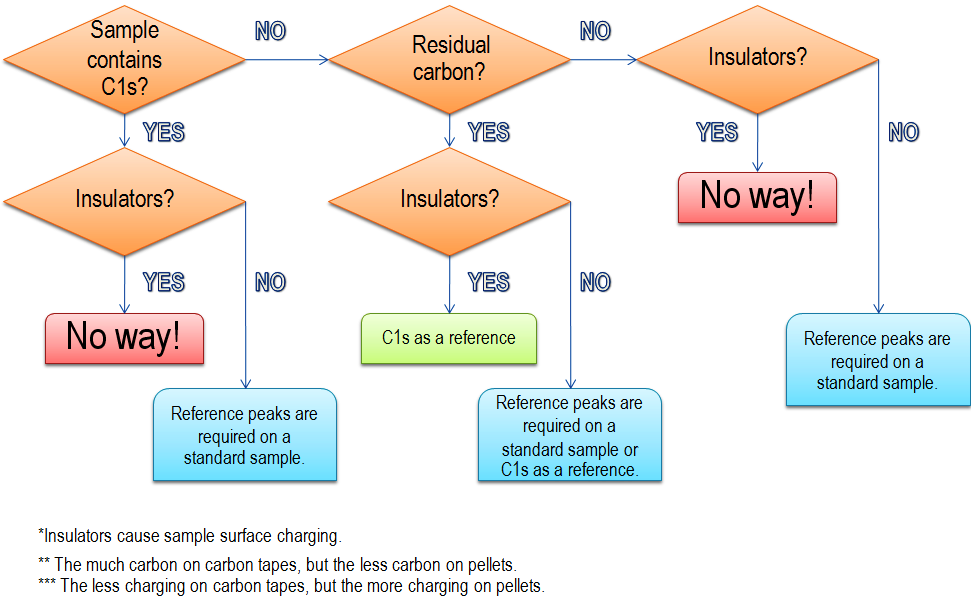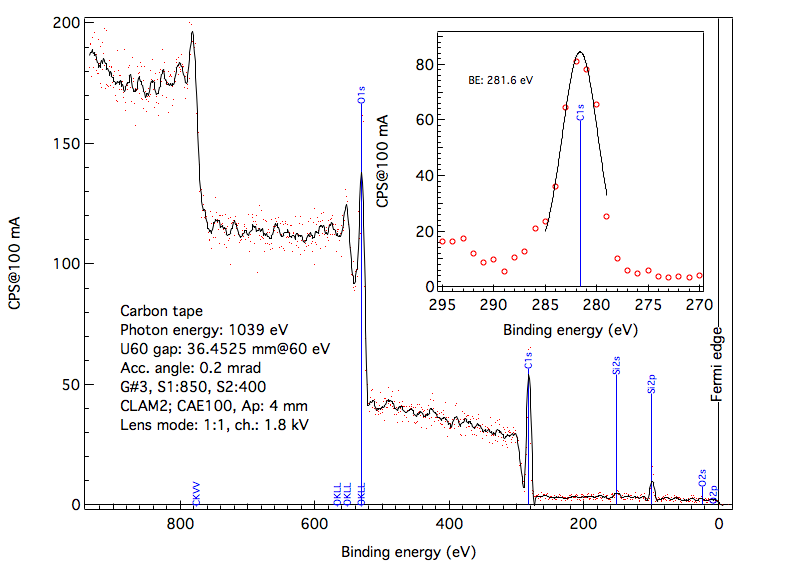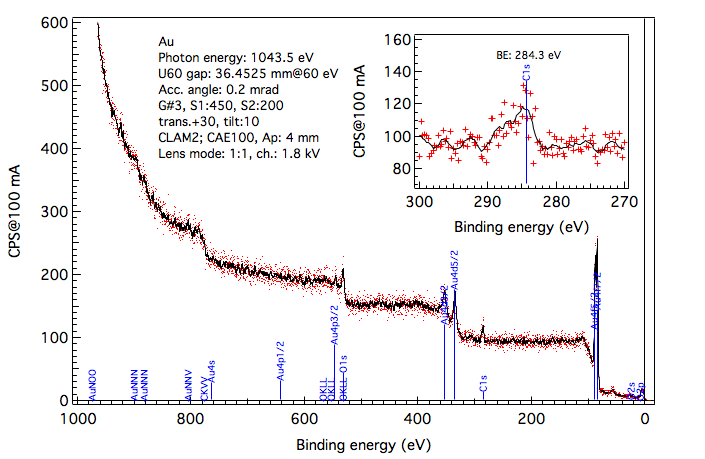How do you know a binding energy of peaks in your spectra?

In materials science, a binding energy of core electrons is a key parameter to characterize the materials, because the binding energy represents the chemical state as well as element of atom. In the core-level spectroscopy, the binding energy is a measurable parameter defined in a difference from the Fermi energy to a peak energy and represents an energy of system involved in the photoemission process. Therefore, in a simple way, XPS enables us to identify materials and its properties through the binding energy in the standard reference sample.

No serious problem is in metallic sample, where the Fermi energy is defined by measuring the Fermi cutoff located in the top of valence band. If the standard reference sample is available to measure it in XPS, its binding energy can be used as a binding energy reference instead of the Fermi energy. Semiconductor and insulator samples show no clear-cutoff at the Fermi level, so the XPS measurement on a reference peak from the standard sample is always required prior to each target sample measurement.

On the insulator sample measurement, energy shifts are observed in a whole photoelectron spectrum being due to a negatively charged sample surface. This charging effect might be different between the insulator and standard reference samples, even though it is reduced with either electron showers generated by the electron flood gun on the sample surfaces or decrease in the incoming photon fluence by using the small slit width or higher photon energy. Therefore, the residual carbon 1s peak measurement is crucial to calibrate the binding energies on the charging samples. Residual carbon-speicies 1s binding energy is 284.3 eV, while those in specific materials are also listed in the following link;If the carbon 1s peak is a primary target to study materials, the sample must be conductive. If the sample is charging, the residual carbon should not be removed from sample surfaces by ion sputtering.

The charging effect is confirmed with electron showers, which make highly resolved peak structures. The decrease of the photon fluence results in the low photonelectron yield.

The XPS spectral profile shows both Auger and photoemission peaks, so the photon energy should be tuned to clarify the all peaks from characteristic elements in your samples prior to the binding energy calibration. The kinetic energy of Auger electrons does not depend on the inident photon and electron enegies. In the XPS spectra with different photon enegies, the Auger peaks remain at the same kinetic energy positions, while the photoemission peaks from core electrons shift as a function of photon energy. However, the binding energy of photoemission peaks is independent of the photon energy.

The photoelectron kinetic energy (KE) excited by a photon energy (PE) is measured from a reference from the spectrometer workfunction (
WF), so the binding energy (BE) is formulated as the following equation;

PE = BE + (KE + WF).

In general, PE and WF are not exactly well-known, because they slightly change depending on the instrumental environment. Therefore, this equation can be employed in a rough estimation of PE, KE and so BE. In practical use, the equation is modifed as below;

DE = PE - RB - (RK + WF),

BE = PE - (KE + WF) - DE.

Here, RB and RK represent the binding and kinetic energies of the standard reference sample or carbon peak to evaluate the factor of DE. This DE can be used to calibrate the binding energy of samples. DE should be obtained in each XPS measurement to compensate the instabilities in PE and WF.

The standard data on the chemical shifts are available in several web described below. However, one takes into account the fact that core-level shifts on surface and its impurities are not the same as those in bulk.Some examples are shown in the figures below. Conductive carbon tape can be used as a reference for conductive samples.Synchrotron Light Research Institute,